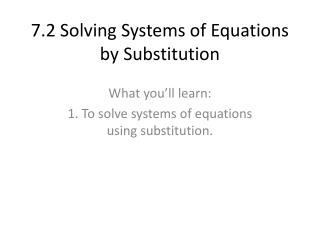DownloadDownload Presentation7.2 Solving Systems of Equations by Substitution

# 7.2 Solving Systems of Equations by Substitution

Télécharger la présentation## 7.2 Solving Systems of Equations by Substitution

- - - - - - - - - - - - - - - - - - - - - - - - - - - E N D - - - - - - - - - - - - - - - - - - - - - - - - - - -
##### Presentation Transcript

1. 7.2 Solving Systems of Equations by Substitution What you’ll learn: 1. To solve systems of equations using substitution.

2. To solve a system by substitution: • Solve one equation for one of the variables (x or y.) • Plug into the other equation what that variable is equal to. • Solve that equation. • If you found y, plug y into the other equation to find x and vice-versa. (Remember that the solution should be an ordered pair, so you have to find x and y. • If your variable cancels and a false statement remains, there is no solution. If your variable cancels and a true statement remains, the solution is “infinitely many.”

3. Example: • x=4y 4x-y=75 4(4y)-y=75 16y-y=75 15y=75 y=5 x=4y x=4(5) x=20 Solution: (20,5) Check: 20=4(5) 4(20)-5=75 • 4x+y=12 -2x-3y=14 y=-4x+12 -2x-3(-4x+12)=14 -2x+12x-36=14 10x-36=14 10x=50 x=5 y=-4x+12 y=-4(5)+12 y=-20+12 y=-8 solution: (5,-8) Check: 4(5)+(-8)=12 -2(5)-3(-8)=14

4. More Examples • 2x+2y=8 x+y=-2 Solve 2nd equ. for y y=-x-2 Plug into 1st equ. in place of y 2x+2(-x-2)=8 2x-2x-4=8 -4=8 No solution • c-4d=1 2c-8d=2 Solve 1st equ. for c c=4d+1 Plug into 2nd equ 2(4d+1)-8d=2 8d+2-8d=2 2=2 Infinitely many

5. Word Problems Write a system and solve: Tickets to a movie cost \$7.25 for adults and \$5.50 for students. A group of friends purchased 8 tickets for \$52.75. How many adults tickets and student tickets were purchased? x=adults tickets, y=student tickets x+y=8 7.25x+5.50y=52.75 y=-x+8 7.25x+5.50(-x+8)=52.75 7.25x-5.50x+44=52.75 1.75x=8.75 x=5, y=3